Courses

# Doc: Electrostatic Potential due to a Point Charge Class 12 Notes | EduRev

## Class 12 : Doc: Electrostatic Potential due to a Point Charge Class 12 Notes | EduRev

The document Doc: Electrostatic Potential due to a Point Charge Class 12 Notes | EduRev is a part of the Class 12 Course Physics Class 12.
All you need of Class 12 at this link: Class 12

Electric Potential Due to a Point Charge

The electric potential of a point charge Q is given by V = kQ/r.

Key Points

• Recall that the electric potential is defined as the potential energy per unit charge, i.e. V = PE/q
• The potential difference between two points ΔV is often called the voltage and is given by  ΔV = VB − VA = ΔPE/q .
• The potential at an infinite distance is often taken to be zero.
• The case of the electric potential generated by a point charge is important because it is a case that is often encountered. A spherical sphere of charge creates an external field just like a point charge, for example.
• The equation for the electric potential due to a point charge is V = kQ/r , where k is a constant equal to 9.0×109 N⋅m2/C2.

Key Terms

Electric potential: The potential energy per unit charge at a point in a static electric field; voltage.
Voltage: The amount of electrostatic potential between two points in space.

Recall that the electric potential is defined as the electric potential energy per unit charge

V = PE/q

The electric potential tells you how much potential energy a single point charge at a given location will have. The electric potential at a point is equal to the electric potential energy (measured in joules) of any charged particle at that location divided by the charge (measured in coulombs) of the particle. Since the charge of the test particle has been divided out, the electric potential is a “property” related only to the electric field itself and not the test particle. Another way of saying this is that because PE is dependent on q, the q in the above equation will cancel out, so V is not dependent on q.

The potential difference between two points ΔV is often called the voltage and is given by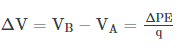Point Charges
Point charges, such as electrons, are among the fundamental building blocks of matter. Furthermore, spherical charge distributions (like on a metal sphere, see figure below) create external electric fields exactly like a point charge. The electric potential due to a point charge is, thus, a case we need to consider. Using calculus to find the work needed to move a test charge q from a large distance away to a distance of r from a point charge Q, and noting the connection between work and potential (W=–qΔV), it can be shown that the electric potential V of a point charge is
V = kQ/r (point charge)
where k is a constant equal to 9.0×109 N⋅m2/C2 .
The potential at infinity is chosen to be zero. Thus V for a point charge decreases with distance, whereas E for a point charge decreases with distance squared: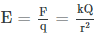The electric potential is a scalar while the electric field is a vector. Note the symmetry between electric potential and gravitational potential – both drop off as a function of distance to the first power, while both the electric and gravitational fields drop off as a function of distance to the second power.

Superposition of Electric Potential

To find the total electric potential due to a system of point charges, one adds the individual voltages as numbers.

Key Points

• The electric potential V is a scalar and has no direction, whereas the electric field E is a vector.
• To find the voltage due to a combination of point charges, you add the individual voltages as numbers. So for example, in the electric potential at point L is the sum of the potential contributions from charges Q1, Q2, Q3, Q4, and Q5 so that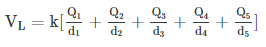.

• To find the total electric field, you must add the individual fields as vectors, taking magnitude and direction into account. This is consistent with the fact that V is closely associated with energy, a scalar, whereas E is closely associated with force, a vector.
• The summing of all voltage contributions to find the total potential field is called the superposition of electric potential. It is much easier to sum scalars than vectors, so often the preferred method for solving problems with electric fields involves the summing of voltages.

Key Terms

Vector: A directed quantity, one with both magnitude and direction; the between two points.
Scalar: A quantity that has magnitude but not direction; compare vector.
Superposition: The summing of two or more field contributions occupying the same space.

Superposition of Electric Potential
We’ve seen that the electric potential is defined as the amount of potential energy per unit charge a test particle has at a given location in an electric field, i.e.

V = PE/q

We’ve also seen that the electric potential due to a point charge is
where k is a constant equal to 9.0×109 N⋅m2/C2. The equation for the electric potential of a point charge looks similar to the equation for the electric field generated for a point particle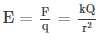with the difference that the electric field drops off with the square of the distance while the potential drops off linearly with distance. This is analogous to the relationship between the gravitational field and the gravitational potential.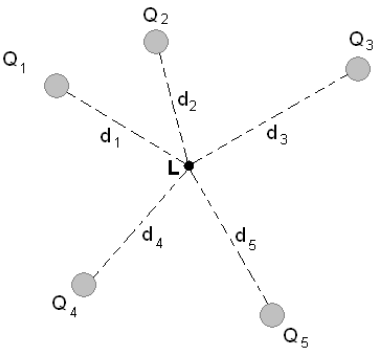Superposition of Electric Potential: The electric potential at point L is the sum of voltages from each point charge (scalars).

Recall that the electric potential V is a scalar and has no direction, whereas the electric field E is a vector. To find the voltage due to a combination of point charges, you add the individual voltages as numbers. So for example, in the figure above the electric potential at point L is the sum of the potential contributions from charges Q1, Q2, Q3, Q4, and Q5 so thatTo find the total electric field, you must add the individual fields as vectors, taking magnitude and direction into account. This is consistent with the fact that V is closely associated with energy, a scalar, whereas E is closely associated with force, a vector.

The summing of all voltage contributions to find the total potential field is called the superposition of electric potential. Summing voltages rather than summing the electric simplifies calculations significantly, since addition of potential scalar fields is much easier than addition of the electric vector fields. Note that there are cases where you might need to sum potential contributions from sources other than point charges; however, that is beyond the scope of this section.

Example:
What Voltage Is Produced by a Small Charge on a Metal Sphere?
Charges in static electricity are typically in the nanocoulomb (nC) to microcoulomb (µC) range. What is the voltage 5.00 cm away from the center of a 1-cm diameter metal sphere that has a −3.00nC static charge?

Strategy
As we have discussed in Electric Charge and Electric Field, charge on a metal sphere spreads out uniformly and produces a field like that of a point charge located at its center. Thus we can find the voltage using the equation V=kQ/r.
Solution
Entering known values into the expression for the potential of a point charge, we obtain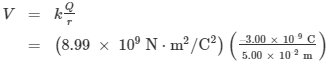–539 V.

Discussion
The negative value for voltage means a positive charge would be attracted from a larger distance, since the potential is lower—more negative—than at larger distances. Conversely, a negative charge would be repelled, as expected.

What Is the Excess Charge on a Van de Graaff Generator

A demonstration Van de Graaff generator has a 25.0 cm diameter metal sphere that produces a voltage of 100 kV near its surface (see Figure 2.11). What excess charge resides on the sphere? Assume that each numerical value here is shown with three significant figures.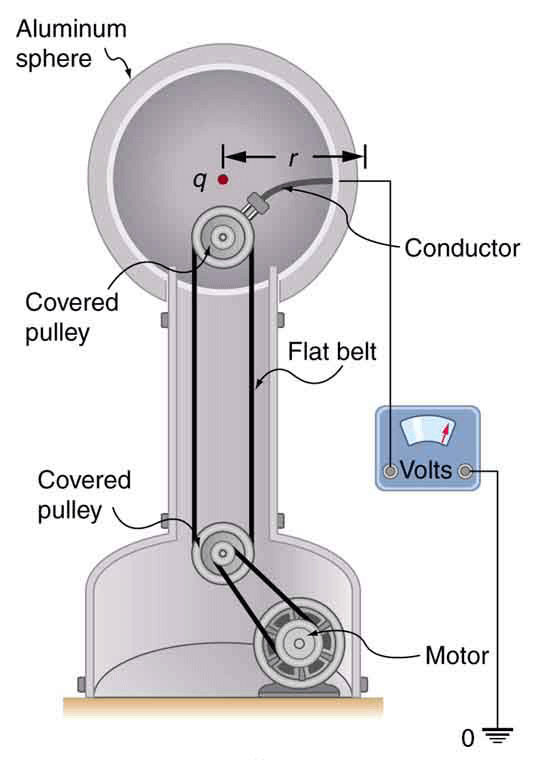Figure 2.11 The voltage of this demonstration Van de Graaff generator is measured between the charged sphere and ground. Earth’s potential is taken to be zero as a reference. The potential of the charged conducting sphere is the same as that of an equal point charge at its center.

Strategy
The potential on the surface will be the same as that of a point charge at the center of the sphere, 12.5 cm away. The radius of the sphere is 12.5 cm. We can thus determine the excess charge using the equation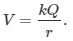Solution
Solving for Q and entering known values gives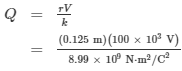= 1.39×10–6 C = 1.39 µC.

Discussion
This is a relatively small charge, but it produces a rather large voltage. We have another indication here that it is difficult to store isolated charges.
The voltages in both of these examples could be measured with a meter that compares the measured potential with ground potential. Ground potential is often taken to be zero—instead of taking the potential at infinity to be zero. It is the potential difference between two points that is of importance, and very often there is a tacit assumption that some reference point, such as Earth or a very distant point, is at zero potential. As noted in Electric Potential Energy: Potential Difference, this is analogous to taking sea level as h=0 when considering gravitational potential energy, PEg=mgh.

Offer running on EduRev: Apply code STAYHOME200 to get INR 200 off on our premium plan EduRev Infinity!

,

,

,

,

,

,

,

,

,

,

,

,

,

,

,

,

,

,

,

,

,

;